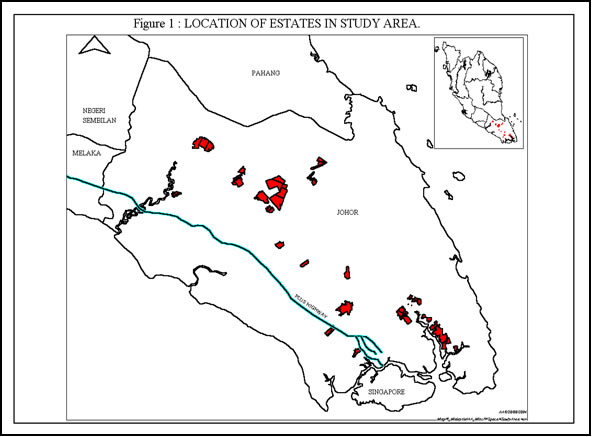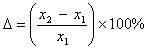# Changes in Soil Properties: Materials and Methods

The data used in this paper were extracted from long-term soil analysis results of samples collected from 21 estates from 1967 to 2000. The estates were located from northern to southern Johor along the central belt (Figure 1). They covered over 37,000 ha, of which data from approximately 13,000 ha were used. Only analysis data of Ultisols from Johor were reported in this paper and they encompassed 26 soil series, which were mostly developed over igneous rocks, sedimentary rocks and riverine alluvium. Examples of soils in the study area would include Rengam series (deep coarse sandy clay soil derived from granite), Bungor series (deep fine sandy clay soil derived from sandstone/shale) and Holyrood series (deep coarse sandy clay loam soil derived from sub-recent alluvium).Sampling Method

36 soil-sampling sites were randomly located in a representative area of each field. At each sampling site, soil samples were collected from two micro-sites of palm circles (PC) and interrows (IR) using a 2.5 cm diameter screw auger. At each micro-site, soil samples from two depths of 0 – 15 cm and 15 – 45 cm were extracted. This gave four distinct soil samples (two micro-sites x two depths) for each field, which were each bulked from the soil samples taken from the 36 sampling sites.

Chemical Analysis

Each soil sample was analyzed for some or all of the soil chemical properties listed in Table 1. We also restricted the data set to soil samples where they were collected and analyzed at the same time for two different soil depths and/or sites in each field. Thus, the number of samples available varied among the soil chemical properties (Table 1).

Soil pH was determined in the supernatent suspension of 1:2.5 soil: water. Organic carbon was analysed by the Walkey-Black procedure while total N was extracted using micro-Kjeldahl method. Total P was extracted using 6M HCl whereas available P followed Bray No. 2 procedure. Exchangeable K, Mg and Ca were extracted by leaching the soil sample with 1 M ammonium acetate solution at pH 7.0. K was then determined using flame photometer while Mg and Ca were determined using atomic absorption spectrophotometer. Details of the above analytical methods can be found in Moris and Mohinder (1980).

Table 1: Number of soil samples analyzed for each soil chemical property

 Number of soil samples analyzed IR PC Soil chemical properties 0-15 cm 15-45 cm 0-15 cm 15-45 cm Total pH 372 372 372 372 1488 Organic C (%) 599 599 – – 1198 Total N (%) 599 599 – – 1198 Total P (mg/kg) 263 263 263 263 1052 Bray-2 P (mg/kg) 400 400 400 400 1600 Exch. K (cmol/kg) 412 412 412 412 1648 Exch. Mg (cmol/kg) 376 376 376 376 1504

Soil Nutrient Changes

Quantifying the rates of change in soil chemical properties for different soils and climate is necessary to reckon if any, the soil fertility decline as very few studies have been conducted in which these rates were calculated (Hartemink, 2003). In this paper, the rate of change was calculated for each soil chemical property as follows (Hartemink, 2003):where Δ = the rate of change in %
1 = the initial value of the variable at sampling time 1
2 = the final value of the variable at sampling time 2

The soil samples where 1 and 2 were determined came from the same field. The period between the soil samplings 2– 1varied from one to 13 years.

Statistical Analysis

The main descriptive statistics such as mean, median, minimum, maximum, standard deviation, standard error of mean were calculated to examine the means and distributions of the initial value (1) and change (%) for each soil chemical property (Appendices 1a to 1d).

Since the rate of change (%) for each soil chemical property was not normally distributed, non-parametric statistics and median were used to analyze the data. Wilcoxon’s signed rank test (Steel and Torrie, 1981) was used to investigate the differences in the rate of change (%) of each soil chemical property between the two sites of IR and PC, and between the two soil depths (0-15 cm vs. 15-45 cm).

In the Wilcoxon’s signed rank test, the sample size (Table 2) did not equal to half the number of soil samples collected (Table 1). This was because in calculating the rate of change (%), we took two soil samples (1 and 2) that were collected from the same field but at different sampling periods (t1 and t2). This implied that if a field was sampled twice at two different sampling periods, then the sample size equaled to one. On the other hand, if a field was sampled three times, then the sample size equaled to three, i.e. the rates of change (%) were calculated from1 and 22 and 3 as well as 1 and 3. The same applies for other soil samples taken at different periods from the same fields i.e. four sampling periods would yield a sample size of four.